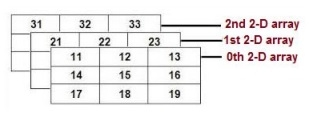# Initialization of a multidimensional arrays in C/C++

In multidimensional array, the array should have dimension more that 1. The following diagram shows the memory allocation strategy for a multidimensional array with dimension 3 x 3 x 3.This is a C++ program to initialize a multidimensional array.

## Algorithm

Begin
Initialize the elements of a multidimensional array.
Print the size of the array.
Display the content of the array.
End

## Example

#include<iostream>
using namespace std;
int main()
{
int r, c;
int a[] = {{3,1},{7,6}};
cout<< "Size of the Array:"<<sizeof(a)<<"\n";
cout<< "Content of the Array:"<<sizeof(a)<<"\n";
for(r=0; r<2; r++) {
for(c=0; c<2; c++) {
cout << " " << a[r][c];
}
cout << "\n";
}
return 0;
}

## Output

Size of the Array:16
Content of the Array:16
3 1
7 6

Updated on: 30-Jul-2019

95 Views